Optics Exam1 and Problem Solutions

Optics Exam1 and  Problem Solutions

1. In the picture given below, you see object placed at point A and it's motion at point A'. If we rotate plane mirror 300 in clockwise direction, find the final location of image of the object.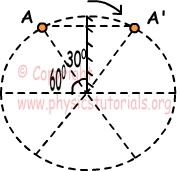If plane mirror rotates 300, then image of the object rotates 600. (We have learned this rule in content part. If plane mirror rotates α, then image of the object rotates 2α). Thus, image of the object is;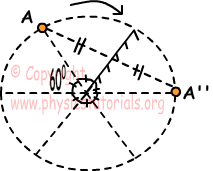2. Rays coming from the object A, first reflects from mirror 1 and then reflects from mirror 2. Draw the images of this object.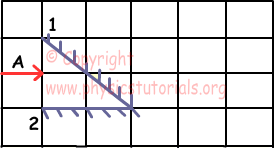Image of the object is placed symmetrically behind the plane mirror.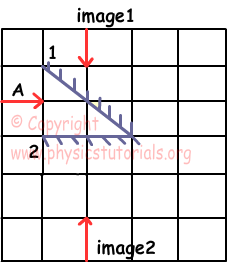3.  Rays coming from the object AB, first reflects from mirror 1 and then reflects from mirror 2. Draw the images of this object in mirror 2.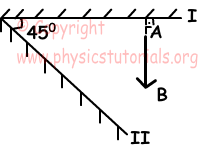Rays coming from object AB reflects from mirror one and image A'B' is formed behind the mirror I. Rays coming from A'B' reflects from mirror II and image A''B'' is formed behind mirror II.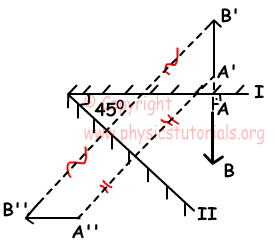4. If velocities of the mirror and object with respect to ground are V, and 2V, find the velocity of the image with respect to ground.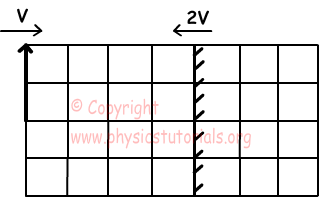Object, mirror and image of the object after t seconds are shown in the figure below. We find the velocity of image using this picture.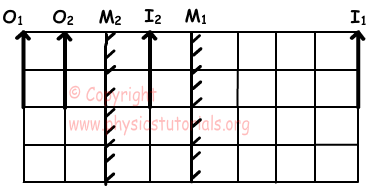As you can see on the picture given above, image of the object change 5 unit distance, if we say V to 1unit change in t seconds, velocity of the image becomes 5V.

5. An observer looks at the mirror 1, find the image of the point source with respect to observer.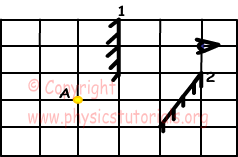Rays coming from the point source do not hit first mirror directly. They reflect from second mirror and then come to the first mirror. Thus, image of the second mirror becomes object of the first mirror. We draw image of the point source like in the picture given below.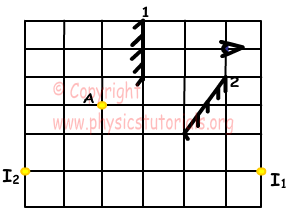Author: